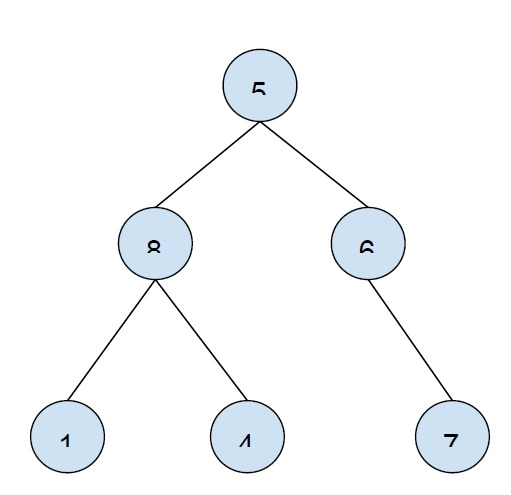# Print all leaf nodes of a binary tree from right to left in C++

In this problem, we are given a binary tree and we have to print all leaf nodes of the binary tree from right to left.

Let’s take an example to understand the problem

InputOutput − 7 4 1

To solve this problem, we will have to traverse the binary tree. This traversal can be done in two ways −

Preorder traversal − This traversal uses recursion. Here, we will traverse, root then left and then right subtree. If we encounter a leaf node then we will print it, else we check for children of the node and explore them to find leaf node.

## Example

Program to show the implementation of our solution −

Live Demo

#include <iostream>
using namespace std;
struct Node {
int data;
struct Node *left, *right;
};
Node* insertNode(int data) {
Node* temp = new Node;
temp->data = data;
temp->left = temp->right = NULL;
return temp;
}
void findLeafNode(Node* root) {
if (!root)
return;
if (!root->left && !root->right) {
cout<<root->data<<"\t";
return;
}
if (root->right)
findLeafNode(root->right);
if (root->left)
findLeafNode(root->left);
}
int main() {
Node* root = insertNode(21);
root->left = insertNode(5);
root->right = insertNode(11);
root->left->left = insertNode(8);
root->left->right = insertNode(98);
root->right->left = insertNode(2);
root->right->right = insertNode(8);
cout<<"Leaf nodes of the tree from right to left are:\n";
findLeafNode(root);
return 0;
}

## Output

Leaf nodes of the tree from right to left are −
18 2 98 8

Postorder Traversal − This traversal to find the leaf node will use iteration. We will use a stack to store data and traverse the tree in a postorder manner (first right subtree then left subtree and then root) and print leaf nodes.

## Example

Program to show the implementation of our solution −

Live Demo

#include<bits/stdc++.h>
using namespace std;
struct Node {
Node* left;
Node* right;
int data;
};
Node* insertNode(int key) {
Node* node = new Node();
node->left = node->right = NULL;
node->data = key;
return node;
}
void findLeafNode(Node* tree) {
stack<Node*> treeStack;
while (1) {
if (tree) {
treeStack.push(tree);
tree = tree->right;
} else {
if (treeStack.empty())
break;
else {
if (treeStack.top()->left == NULL) {
tree = treeStack.top();
treeStack.pop();
if (tree->right == NULL)
cout<<tree->data<<"\t";
}
while (tree == treeStack.top()->left) {
tree = treeStack.top();
treeStack.pop();
if (treeStack.empty())
break;
}
if (!treeStack.empty())
tree = treeStack.top()->left;
else
tree = NULL;
}
}
}
}
int main(){
Node* root = insertNode(21);
root->left = insertNode(5);
root->right = insertNode(11);
root->left->left = insertNode(8);
root->left->right = insertNode(98);
root->right->left = insertNode(2);
root->right->right = insertNode(18);
cout<<"Leaf nodes of the tree from right to left are:\n";
findLeafNode(root);
return 0;
}

## Output

Leaf nodes of the tree from right to left are −
18 2 98 8